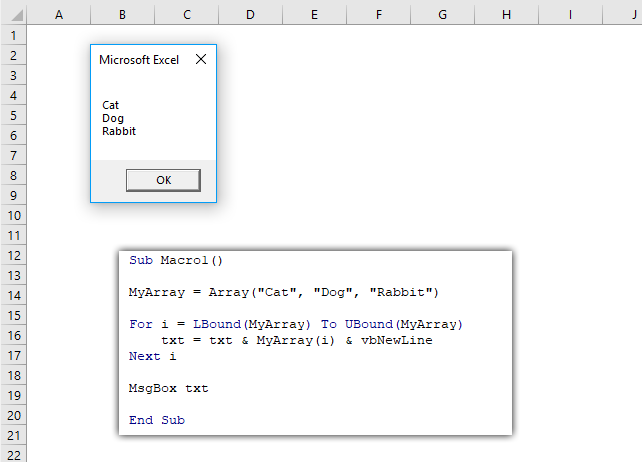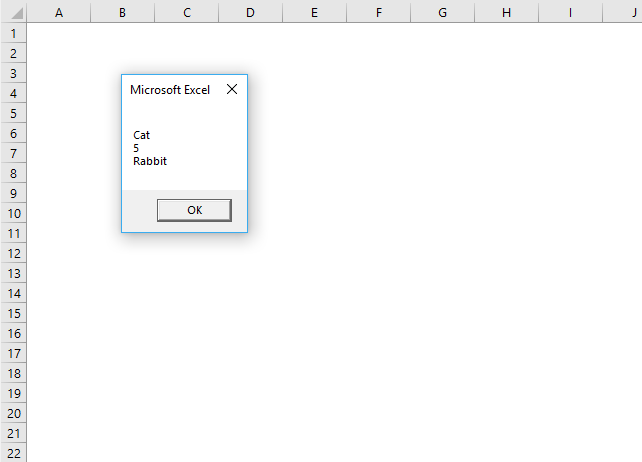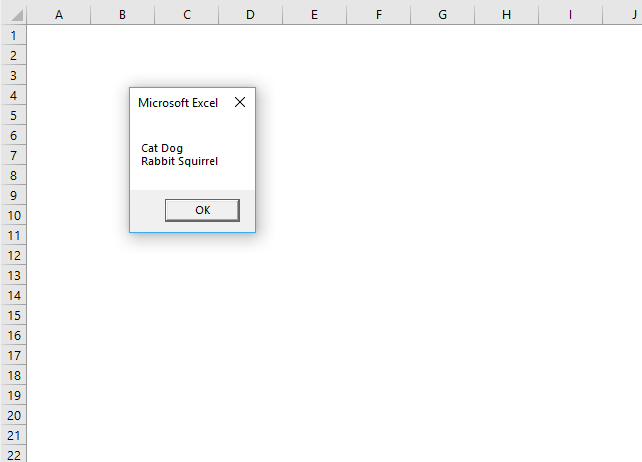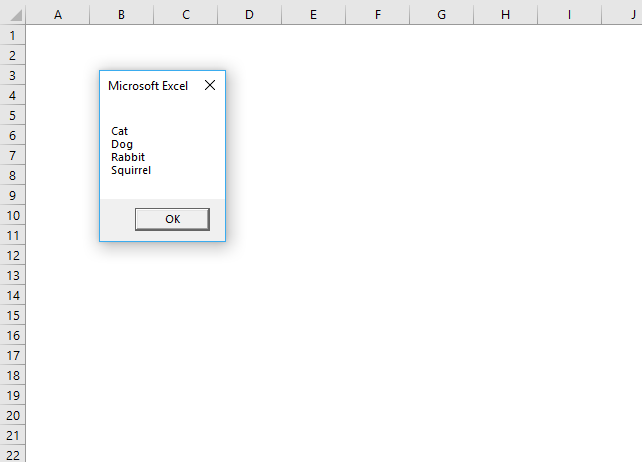Author: Oscar Cronquist Article last updated on March 15, 2022The ARRAY function creates a Variant variable containing array values.

Array(arglist)

## 2. Array Function Arguments

 arglist A list of values. Text strings must have a beginning and ending double quote. The values must be separated by a comma. If omitted a zero-length array is created.

## 3. Array Function example

```'Name macro
Sub Macro1()

'Populate array variable
MyArray = Array("Cat", "Dog", "Rabbit")

'For Next statement using lower and upper boundaries of the array variable
For i = LBound(MyArray) To UBound(MyArray)

'Save array value to variable txt and a new line
txt = txt & MyArray(i) & vbNewLine

'Continue with next value
Next i

'Show message box containing contents of variable txt
MsgBox txt

'Exit macro
End Sub
```

The macro above populates variable MyArray with values Cat, Dog and Rabbit.

A FOR ... NEXT statement loops through the array variable using the Lbound and Ubound function to determine the lower and upper limit of the array.

An ampersand then concatenates the values and lastly, the msgbox shows the concatenated string.

## 4. Alternative way to populate an array variable

The following macro demonstrates another way to create an array variable. [arglist] means evaluate.```'Name macro
Sub Macro2()

'Populate array variable using brackets and curly brackets
MyArray = [{"Cat", 5, "Rabbit"}]

'For Next statement using lower and upper boundaries of the array variable
For i = LBound(MyArray) To UBound(MyArray)

'Save array value to variable txt and a new line
txt = txt & MyArray(i) & vbNewLine

'Continue with next value
Next i

'Show message box containing contents of variable txt
MsgBox txt

'Exit macro
End Sub
```

## 5. Populate a two-dimensional array variable

This macro creates array variable MyArray with values in two dimensions.```'Name macro
Sub Macro3()

'Populate array variable using two Array functions
MyArray = Array(Array("Cat", "Dog"), Array("Rabbit", "Squirrel"))

'For Next statement for rows
For r = 0 To 1

'For Next statement for columns
For c = 0 To 1

'Save array value to variable txt and a new line
txt = txt & MyArray(r)(c) & " "
'Continue with next value
Next c

'Add a new line to variable txt
txt = txt & vbNewLine

'Continue with next value
Next r

'Show message box containing contents of variable txt
MsgBox txt

'Exit macro
End Sub
```

## 6. Alternative way to populate a two-dimensional array variable

This macro populates array variable MyArray with values in two dimensions using the evaluate characters.```'Name macro
Sub Macro4()

'Populate array variable using brackets and curly brackets
MyArray = [{"Cat", "Dog"; "Rabbit", "Squirrel"}]

'For Next statement for rows using lower and upper boundaries
For r = LBound(MyArray, 1) To UBound(MyArray, 1)

'For Next statement for columns using lower and upper boundaries
For c = LBound(MyArray, 2) To UBound(MyArray, 2)

'Save array value to variable txt and a new line
txt = txt & MyArray(r, c) & vbNewLine

'Continue with next value
Next c

'Continue with next value
Next r

'Show message box containing contents of variable txt
MsgBox txt

'Exit macro
End Sub
```